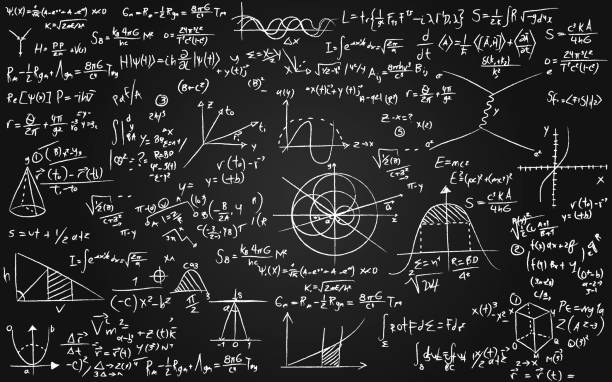iStockMath Equations Written On A Blackboard Stock Illustration - Download Image Now - Mathematical Symbol, Mathematics, Mathematical FormulaDownload this Math Equations Written On A Blackboard vector illustration now. And search more of iStock's library of royalty-free vector art that features Mathematical Symbol graphics available for quick and easy download.Product #:gm1219382595\$33.00iStockIn stock
Only from iStock

# Math equations written on a blackboard stock illustrationMath equations written on a blackboard - mathematics and science concepts

## Description

Math equations written on a blackboard - mathematics and science concepts

3 credits

## Signature

View plans and pricing

Largest size:Vector (EPS) – Scalable to any size
Stock illustration ID:1219382595